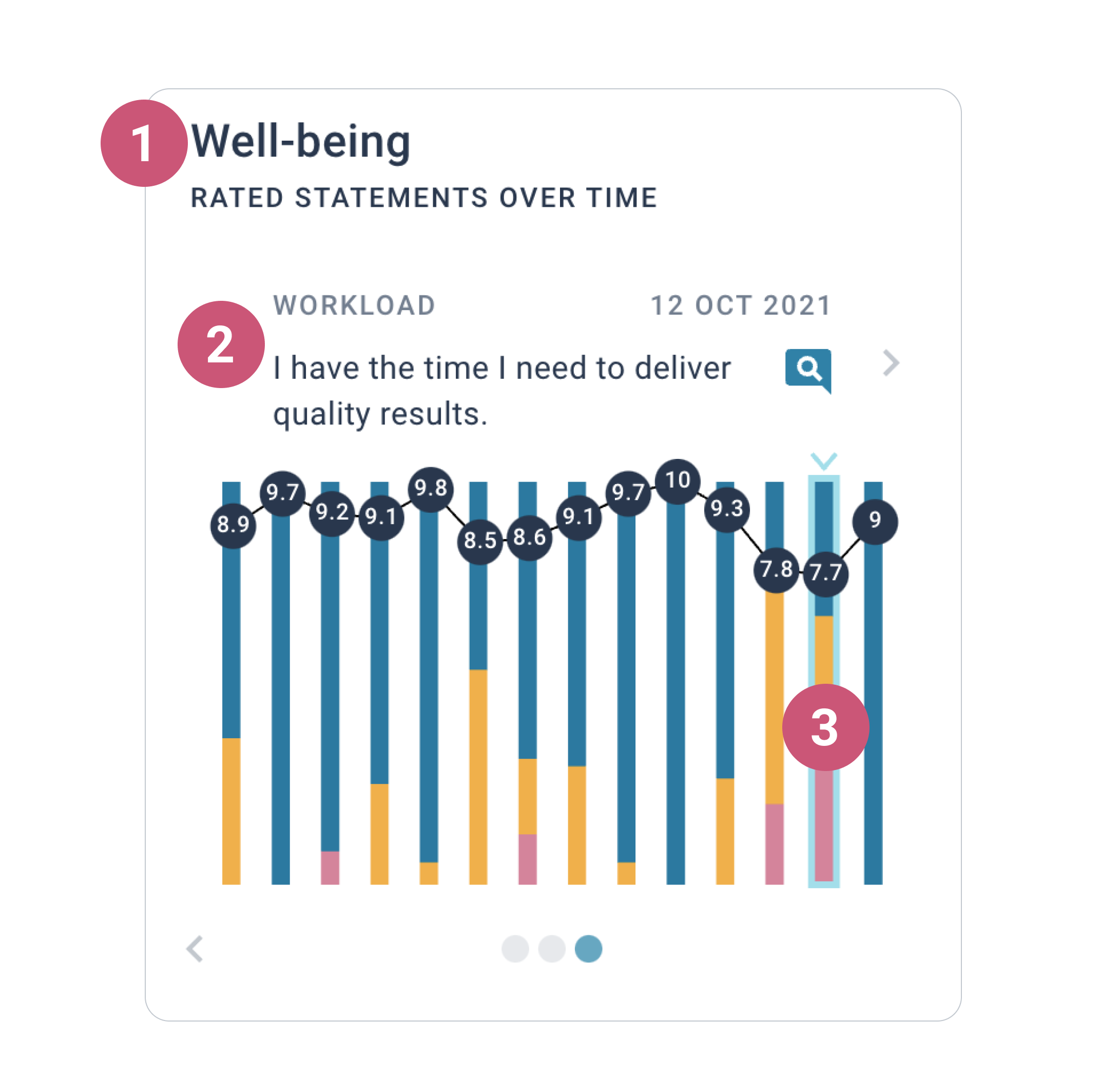1. Help Center
2. How to use Joyous
3. Understanding Insights

Understanding rated statements over time

📺  Watch the video lesson here: Understanding insights

Rated statements over time is an easy way for you to see average scores by rating scale question in a bar graph.

What is shown in rated statements over time

Rated statements over time consist of three key pieces of information.1. The category name.

2. The rated statement details for the selected bar in the chart.

• This includes the rated statement text, the topic, and the date the question was sent.

📝  Note: If the same rating scale question has been asked more than once over time, only the most recent question round will be included in the chart

3. The bar chart.

• The bar chart includes one bar for each rating scale question asked, arranged by the date the question was sent (oldest to newest).
• Within each bar, the percentage distribution of scores by sentiment is displayed.
• The pink portion of the bar shows the percentage of negative scores (0 through 6).
• The yellow portion of the bar shows the percentage of neutral scores (7 or 8).
• The blue portion of the bar shows the percentage of positive scores (9 or 10).
• Each bar also displays the average score for that rating scale question.
• We use a simple average to calculate the average score.
• Each individual rating contributes equally to an average score. For example:  (7 + 4 + 10 + 0 + 2 + 5) / 6 = 4.7.
• A line is drawn between each average score to make it easy to see the trend over time for the category, although it's important to bear in mind the score is highly dependent on the rating scale question asked.
• Simply tap or click on a bar to select it. This will allow you to see the rated statement details for the selected bar above the chart.